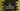# Write a C program to add two distances using structure## Write a C program to add two distances using structure:

This program will show you how to add two distance values using structures. The distance values are represented in feet and inch. The program will take the values as input from the user and find out the sum.

To solve this program using structure, we will create one structure, that can save feet and inch in two different integer variables.

Below is the complete algorithm that this program will use:

### Algorithm to add two distances using structures in C:

• Create one structure that can hold feet and inch values.
• Create two variables of the structure.
• Ask the user to enter the feet and inch values for both distances. Read and store these values in the variables.
• Add the feet and inch values of both distance variables. Store it in a different sum variable.
• Convert the inch to feet. For each inch > 12 in the sum, decrement it by 12 and increment the value of feet by 1.
• Once the conversion ends, print the value of sum.

### C Program:

Below is the complete C program :

``````#include <stdio.h>

struct Distance
{
int feet;
float inch;
} firstDistance, secondDistance, sum;

int main()
{
printf("Enter feet and inch for the first distance with a space : \n");
scanf("%d %f", &firstDistance.feet, &firstDistance.inch);

printf("Enter feet and inch for the second distance with a space : \n");
scanf("%d %f", &secondDistance.feet, &secondDistance.inch);

sum.feet = firstDistance.feet + secondDistance.feet;
sum.inch = firstDistance.inch + secondDistance.inch;

while (sum.inch >= 12)
{
sum.inch = sum.inch - 12;
sum.feet++;
}

printf("Sum is %d feet, %.1f inches\n", sum.feet, sum.inch);
return 0;
}``````

### Explanation:

Here,

• Distance is a structure to hold a distance value. It can hold a integer variable for feet and one floating point value for inch.
• firstDistance and secondDistance variables are created to store the first and the second distance entered by the user. sum is to hold the sum.
• It asks the user to enter the value of both distances one by one. For each distance, it asks to enter the feet and inch values.
• Once the distance values are read, it adds the feet and inch values of both and store it in sum.
• The while loop converts the value of inch to feet. It decrements the inch value to less than 12 and adds them to inch value.

### Sample output:

``````Enter feet and inch for the first distance with a space :
12 30
Enter feet and inch for the second distance with a space :
20 40
Sum is 37 feet, 10.0 inches``````